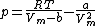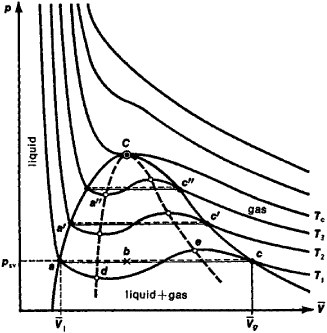# van der Waals equation

(redirected from Van der Waals equation of state)
Also found in: Dictionary.

## van der Waals equation:

see gas lawsgas laws,
physical laws describing the behavior of a gas under various conditions of pressure, volume, and temperature. Experimental results indicate that all real gases behave in approximately the same manner, having their volume reduced by about the same proportion of the
.

## Van der Waals equation

An equation of state of gases and liquids proposed by J. D. van der Waals in 1873 that takes into account the nonzero size of molecules and the attractive forces between them. He expressed the pressure p as a function of the absolute temperature T and the molar volume Vm = V/n, where n is the number of moles of gas molecules in a volume V (see equation below).Here R = 8.3145 J K-1 mol-1 is the universal gas constant, and a and b are parameters that depend on the nature of the gas. Parameter a is a measure of the strength of the attractive forces between the molecules, and b is approximately equal to four times the volume of the molecules in one mole, if those molecules can be represented as elastic spheres. The equation has no rigorous theoretical basis for real molecular systems, but is important because it was the first to take reasonable account of molecular attractions and repulsions, and to emphasize the fact that the intermolecular forces acted in the same way in both gases and liquids. It is accurate enough to account for the fact that all gases have a critical temperature Tc above which they cannot be condensed to a liquid. The expression that follows from this equation is Tc= 8a/27 Rb. See Critical phenomena, Intermolecular forces

In a gas mixture, the parameters a and b are taken to be quadratic functions of the mole fractions of the components since they are supposed to arise from the interaction of the molecules in pairs. The resulting equation for a binary mixture accounts in a qualitative but surprisingly complete way for the many kinds of gas-gas, gas-liquid, and liquid-liquid phase equilibria that have been observed in mixtures.

The equation is too simple to represent quantitatively the behavior of real gases, and so the parameters a and b cannot be determined uniquely; their values depend on the ranges of density and temperature used in their determination. For this reason, the equation now has little practical value, but it remains important for its historical interest and for the concepts that led to its derivation. See Thermodynamic principles

McGraw-Hill Concise Encyclopedia of Physics. © 2002 by The McGraw-Hill Companies, Inc.
The following article is from The Great Soviet Encyclopedia (1979). It might be outdated or ideologically biased.

## Van Der Waals’ Equation

one of the first equations of the state of a real gas; proposed by the Dutch physicist J. D. van der Waals in 1873:

(p +a/⊽ 2)(⊽ - b) = RT

Here p is the gas pressure, T is the gas temperature, ⊽ is the volume of one mole of the substance, R is the universal gas constant, and a and b are constants that account for the deviation of the properties of a real gas from those of an ideal gas. The term a/⊽ 2, which has the dimension of pressure, accounts for the attraction between the molecules of the gas owing to van der Waals’ forces. The constant b is the correction for the intrinsic volume of the gas molecules and allows for the repulsion of molecules at close distances. The constants a and b are usually determined from experimental data. For large values of ⊽ it is possible to ignore both corrections, and van der Waals’ equation is converted into the equation of the state of an ideal gas.

Van der Waals’ equation is approximate and quantitatively describes the properties of real gases only in regions of high temperatures and low pressures. However, it permits the qualitative description of the behavior of a gas at high pressures, the condensation of a gas into a liquid, and the critical state.Figure 1. Diagram of the state of a substance in volume-pressure (⊽,p) coordinates: T1, T2, T3, and Tc are isotherms calculated according to van der Waals’ equation; C is the critical point. The line dCe (spinodal) outlines the region of unstable states.

Figure 1 shows a family of isotherms (curves of the dependence of p on ⊽ at constant temperature) calculated according to van der Waals’ equation. This equation, which is cubic with respect to ⊽ has three roots. At low temperatures all three roots are real, but above a specific temperature Tc , called the critical temperature, only one real root remains. Physically, this means that for T > Tc the substance can exist in only one state (the-gaseous), and below Tc, in three states (two stable—liquid 1 and gaseous Vg—and one unstable). This is expressed graphically in the following way: for T < TC the isotherm has three points of intersection with the straight line ac, parallel to the volume axis. The points of the straight line ac correspond to the equilibrium of liquid and its saturated vapor. Under the equilibrium conditions— for example, in the state corresponding to point b —the relative amounts of liquid and vapor are determined by the ratio of the segments bc/ba (the “rule of moments”). The saturated vapor pressure psy and the volume interval from 1 to ⊽g correspond to the phase equilibrium at a specific temperature. At lower pressures (to the right of g), the isotherm characterizes the properties of the gas. To the left, the almost vertical part of the isotherm indicates a very low compressibility of the liquid. Segments ad and ec belong to superheated liquid and supercooled vapor respectively (metastable states). Segment de is physically unrealized because here there is a volume increase with an increase in pressure. The set of points a , a′ , a″, … and c c′ , c″ , … determines a curve, called a binodal, which outlines the region of simultaneous existence of gas and liquid. At the critical point-C the temperature, pressure, and volume (Tc, pc, and ⊽c) have values that are characteristic of each substance. However, if the relative values T/Tc , p/pc , and ⊽/⊽c are introduced into van der Waals’ equation, then it is possible to obtain the so-called reduced van der Waals’ equation, which is universal— that is, it does not depend on the individual properties of the substance.

### REFERENCES

Shtrauf, E. A. Molekuliarnaia fizika. Leningrad-Moscow, 1949.
Sommerfeld, A. Termodinamika i statisticheskaia fizika. Moscow, 1955. (Translated from German.)
Vukalovich, M. P., and I. I. Novikov. Uravnenie sostoianiia real’nykh gazov. Moscow-Leningrad, 1948.

A. A. LOPATKIN